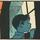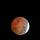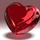## Social Question# How well do you understand weighted averages?

Asked by LostInParadise (31601) May 3rd, 2023

This is something that should be taught in public school. For one thing, a student’s grade is often a weighted average of quizzes, homework, final and class participation.

Here is a non-standard application. There is a Wordle game site that I like, that, after you finish a puzzle, they give the average number of guesses among players for the word. Suppose that the average number of guesses for a word is 4.4. If we make the simplifying assumption that everyone got it in 4 or 5 guesses, what percentage of the players got it in 4 guesses?

Observing members: 0Composing members: 0Since I work with VWAP (volume weighted average price) everyday. I am familiar with the calculation.

However, your question is not about weighted averages, but rather about averages in general. If everyone solved the puzzle in 4 or 5 guesses and the average was 4.4, then 60% guessed 4, and 40% guessed 5.

zenvelo (39284)“Great Answer” (2) Flag as…That is the correct answer. It can be viewed as a weighted average. 4.4 = 0.6×4 + 0.4*5. The case of two values is easy to figure. 4.4 is 40% of the way between 4 and 5, which means that a score of 4 represents 1 – .4 = .6 or 60% of the players.

LostInParadise (31601)“Great Answer” (0) Flag as…Was this not taught in elementary school? I can’t remember ever not knowing this.

Blackwater_Park (8101)“Great Answer” (1) Flag as…LostInParadise (31601)“Great Answer” (0) Flag as…I don’t know what weighted averages is, but that sounds like a very basic percentage question which people learn in school.

smudges (10413)“Great Answer” (0) Flag as…Say you are taking a course with a mid-term, final and three projects. The final is worth 40% the mid-term is worth 30% and the projects are all worth 10%.

Projects: 86, 92, 78
Mid-term: 90
Final: 81

86*.1=8.6
92*.1=9.2
78*.1=7.8
90*.3=27
81*.4=32.4

Blackwater_Park (8101)“Great Answer” (1) Flag as…That is exactly how a weighted average works, The values of .4, .3 and .1 are the weights, and the requirement is that the weights add to 1.0, which is indeed the case.

LostInParadise (31601)“Great Answer” (0) Flag as…While I understand the concept, I get the same answer when I add the numbers together and divide by 5. Maybe there’s a difference in the end result if there are more significant differences in the weighted numbers. ¯\(ツ)

smudges (10413)“Great Answer” (0) Flag as…@smudges consider these scores:
Weight Score weighted score
quiz 5% 100 5
quiz 5% 100 5
project 10% 95 9.5
midterm 30% 85 25.5
final 50% 70 35
average 90 80

This demonstrates how doing well throughout the course until the final would lower a student’s grade to a “B-/C+” level,

zenvelo (39284)“Great Answer” (0) Flag as…^^ Yes, makes sense.

smudges (10413)“Great Answer” (0) Flag as…